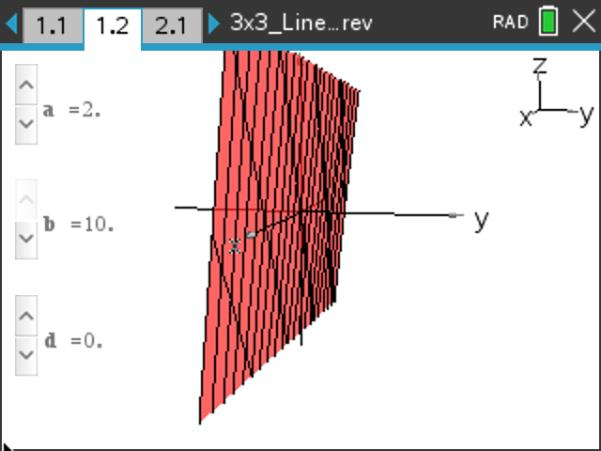••• ##### Device
•TI-Nspire™ CX/CX II
•TI-Nspire™ CX CAS/CX II CAS
• TI-Nspire™ Navigator™
• ##### Software

TI-Nspire™ CX
TI-Nspire™ CX CAS

5.0

• ##### Report an Issue

Precalculus: 3x3 Linear Systems of Equations
by Texas Instruments#### Objectives

• Students will be able to describe the effects of the coefficients of a linear function in three variables on the graph of the function.
• Students will be able to identify the number of solutions to a system of linear equations in three variables by analyzing the graphs of the equations.
• Students will be able to describe the conditions under which a system of linear equations in three variables will have 0, 1, or infinitely many solutions.

#### Vocabulary

• system of equations
• plane
• solution to a system of equations